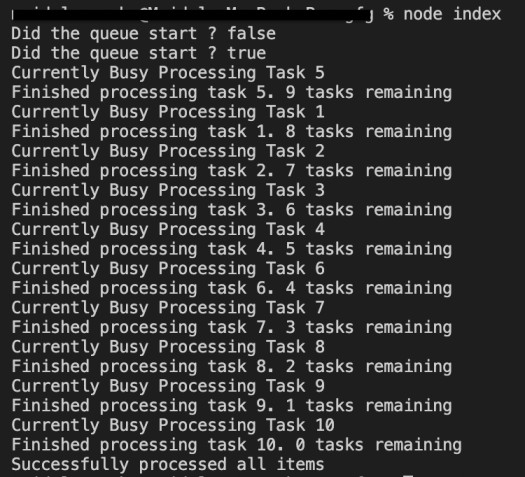GeeksforGeeks App
Open AppBrowser
Continue

## Related Articles

The async module is is designed for working with asynchronous JavaScript in NodeJS. The async.queue returns a queue object which is capable of concurrent processing i.e processing multiple items at a single time.

How to use async.queue?

• Step 1: Create a package.json file. A package.json file is created by the running the following command.

`npm init`
• Step 2: Installing the async module. The async module can be installed using the following command.

`npm i async`
• Step 3: Importing the async module. The async module can be imported using the following command.

`const async = require('async')`
• Step 4: Using the async.queue module. Syntax of async.queue.

`const queue = async.queue('function', 'concurrency value')`

The parameter function is executed on the element added to the queue. The concurrency value tells the queue, the number of elements to be processed at a particular time.

Example: Have a look at the below example for better understanding.

## Javascript

 `// Defining The queue``const queue = async.queue((task, completed) => {``    ``// Here task is the current element being``    ``// processed and completed is the callback function``    ` `    ``console.log(``"Currently Busy Processing Task "` `+ task);``    ` `    ``// Simulating a complex process.``    ``setTimeout(()=>{``        ``// Number of elements to be processed.``        ``const remaining = queue.length();``        ``completed(``null``, {task, remaining});``    ``}, 1000);` `}, 1);` `// The concurrency value is set to one,``// Which means that one element is being``// Processed at a particular time`

Important methods and properties in async.queue:

push(element, callback) :The push method is used to add elements to the tail of the queue. The following code demonstrates how push method works.

1.

## Javascript

 `// Takes in two parameters, the item being pushed and``// the callback function``// Here the item is the element being added``// and other is the callback function with error``// and data property(destructured)` `queue.push(item, (error, {item, remaining}) => {``  ``if``(error){``      ``console.log(`An error occurred ``while` `processing task \${task}`);``  ``} ``else` `{``      ``console.log(`Finished processing task \${task}``             ``. \${remaining} tasks remaining`);``  ``}``});`

1.

length(): The length method returns the number of elements currently present in the queue. The following code demonstrates how the length method works.

1.

## Javascript

 `console.log(queue.length());`

1.

started property: The started property returns a boolean value, indicating whether the queue has started processing the data or not. The following code demonstrates how the started property works.

1.

## Javascript

 `// Returns true if the queue has started processing the data else false``console.log(queue.started)`

1.

unshift(element, callback) :The unshift method is similar to the push method, but the element is added to the head of the queue, indicating that the element to be processed is an important one. The following code demonstrates how the unshift method works :-

1.

## Javascript

 `// Takes in two parameters, the item being pushed``// and the callback function``// Here the item is the element being added``// and other is the callback function with error``// and data property(destructured)` `queue.unshift(item, (error, {item, remaining}) => {``  ``if``(error){``   ``console.log(`An error occurred ``while` `processing task \${task}`);``  ``} ``else` `{``   ``console.log(`Finished processing task \${task}. \${remaining} tasks remaining`);``  ``}``});`

1.

drain() Method : The drain method runs a callback function when the queue is done executing all the tasks. The following code demonstrates how the drain method works.

1.

Note: The drain method only works when the function described is an arrow function.

1.

## Javascript

 `// Executes when the queue is done processing all the items``queue.drain(() => {``    ``console.log(``'Successfully processed all items'``);``})`

1.

pause() Method : The pause method pauses the execution of elements in the queue until the resume function is called. The following code demonstrates how the pause method works.

1.

## Javascript

 `// Pauses the execution of the queue``queue.pause()`

1.

resume() Method: The resume method resumes the execution of elements in the queue. The following code demonstrates how the resume method works.

1.

## Javascript

 `// Resumes the execution of the queue``queue.resume()`

1.

kill() Method: The kill method removes all the elements from the queue, the callback function of the drain method and forces it into idle. The following code demonstrates how the kill method works.

1.

## Javascript

 `// Forces the queue into idle mode``// and removes the drain callback``queue.kill()`

1.

idle() Method: The idle() method return a boolean value, indicating whether the queue is idle or processing something. The following code demonstrates how the idle method works.

1.

## Javascript

 `// Returns whether the queue is idle or not``queue.idle()`

1.

Complete Code: The following code is a complete demonstration of how the async.queue is actually used.

## Javascript

 `// Importing the async module``const async = require(``'async'``);` `// Creating a tasks array``const tasks = [1, 2, 3, 4, 5, 6, 7, 8, 9, 10];` `// Defining the queue``const queue = async.queue((task, completed) => {``    ``console.log(``"Currently Busy Processing Task "` `+ task);``    ` `    ``// Simulating a Complex task``    ``setTimeout(()=>{``        ``// The number of tasks to be processed``        ``const remaining = queue.length();``        ``completed(``null``, {task, remaining});``    ``}, 1000);` `}, 1); ``// The concurrency value is 1`  `// The queue is idle as there are no elements``// for the queue to process``console.log(`Did the queue start ? \${queue.started}`)` `// Adding the each task to the queue``tasks.forEach((task)=>{` `    ``// Adding the 5th task to the head of the``    ``// queue as it is deemed important by us`` ``if``(task == 5){``    ``queue.unshift(task, (error, {task, remaining})=>{``      ``if``(error){``       ``console.log(`An error occurred ``while` `processing task \${task}`);``      ``}``else` `{``       ``console.log(`Finished processing task \${task}. \${remaining} tasks remaining`);``      ``}``    ``})     ``        ``// Adding the task to the tail in the order of their appearance`` ``} ``else` `{``      ``queue.push(task, (error, {task, remaining})=>{``       ``if``(error){``        ``console.log(`An error occurred ``while` `processing task \${task}`);``       ``}``else` `{``        ``console.log(`Finished processing task \${task}. \${remaining} tasks remaining`);``      ``}``      ``})``    ``}``});`  `// Executes the callback when the queue is done processing all the tasks``queue.drain(() => {``    ``console.log(``'Successfully processed all items'``);``})` `// The queue is not idle it is processing the tasks asynchronously``console.log(`Did the queue start ? \${queue.started}`)`

Output:My Personal Notes arrow_drop_up
Related Tutorials